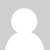### Problem with CT ecs-1030

Hii..
I have the same two CT ecs-1030, and I want to read the current in the cables. In monophase circuit the same current entering is the same out (Kirchhoff's current law). So I have to compare this current and turn off the circuit if the difference of the currents are grater  than 30mA.
I'm using 33ohm burden resistor, 2 10k ohm divide resistor, 10uF capacitor, and Arduino Mega 2560
I get strange values testing one CT.

### Re: Problem with CT ecs-1030

Sorry, but with so little information from you about what is going wrong, nobody will be able to help you. You need to tell us more.### Re: Problem with CT ecs-1030

Sorry men...

I'm doing a project, I have to measure the current in a two wires single-phase motor at the same time. If the difference between these currents are greater than 1 Ampere for a period of 0.5 seconds, I have to turn off the circuit.
Using the example of http://openenergymonitor.org/emon/buildingblocks/arduino-sketch-current-..., I just saw these values ​​when nothing was on the inputs:

2150.94   9.35
2091.40   9.09
2117.81   9.21
2151.48   9.35
2294.22   9.97
2163.98   9.41

Is this normal?

I have 2 ct ecs-1030-L72, I put 120ohm burden resistors as shown in the calculation..

sorry for the English, and I appreciate the help.

### Re: Problem with CT ecs-1030

OK, I think I understand a little.

First, the calibration in that sketch is for a 18 Ω burden resistor. You are using the calibration value 111.1 which is for a 18 Ω burden. For a 120 Ω burden you need a calibration of  16.67. All the calculations that explain how to get that number are here.

So the current you are measuring is approximately 9.3 * 18 / 120 A  =  1.39 A

That is still wrong. We expect to measure a very small current which is not real - it is digital noise from the processor - of perhaps 0.001 x maximum current. In your case, the maximum current is approximately 30 A,  so that would be 15 - 30 mA of "noise".

You need to find out why you are measuring a current when there is none.

If you look at Robin's Tools, try running "MinMaxAndRangeChecker."  If I remember correctly, this will print the values from the Analogue to Digital Converter and let you see exactly that the input is.  With nothing connected, the input you are using should be approximately 512 and not changing by more than 2 or 3 numbers from that.

But you say you have two current transformers. I think you might be doing the measurement the wrong way.  Take a look at how a "Residual Current Device" works and think whether that is doing what you want. (The Wikipedia page has a good explanation.)### Re: Problem with CT ecs-1030

Exactly Robert, I want to make a prototipode of RCD using the arduino.

In RCB regular the two conductors pass the same coil. I thought of making a model that I would examine the current in the two conductors (each CT with a conductor) and compare to make decision making.

Do you think it's the best way?

### Re: Problem with CT ecs-1030

You need to consider the accuracy with which you can measure the two currents if you use two c.t's. You can adjust the calibration at high currents, but at very low currents you are likely to have problems with random or processor noise. That usually amounts to two or three counts peak-peak amplitude. So when you subtract the two currents to get the "leakage" current, you will have 4-6 counts of uncertainty in there.

If you do the difference in the c.t, you need to think about how the c.t. actually works. The flux in the core arises from the difference in the currents - no noise, no linearity problems there. You still have one lot of noise remaining of course - at the ADC input.

I think you should do both and compare the results.

(You must calculate what the noise represents in terms of current using your resistor and calibration values.)### Re: Problem with CT ecs-1030

Should I to change something in the program to put the two sensors?

double Irms = emon1.calcIrms (1480) / / Calculate Irms only
What is this value  1480?

### Re: Problem with CT ecs-1030

Have a look at the comment in the source code of emonLib - "1480" is explained in there.

Remember that emonLib was designed to sample power at intervals and report the value back by radio. What you are trying to do is quite different, so you need to design your hardware and software from the beginning. emonLib will help with some parts but you must not think it will do exactly what you want.